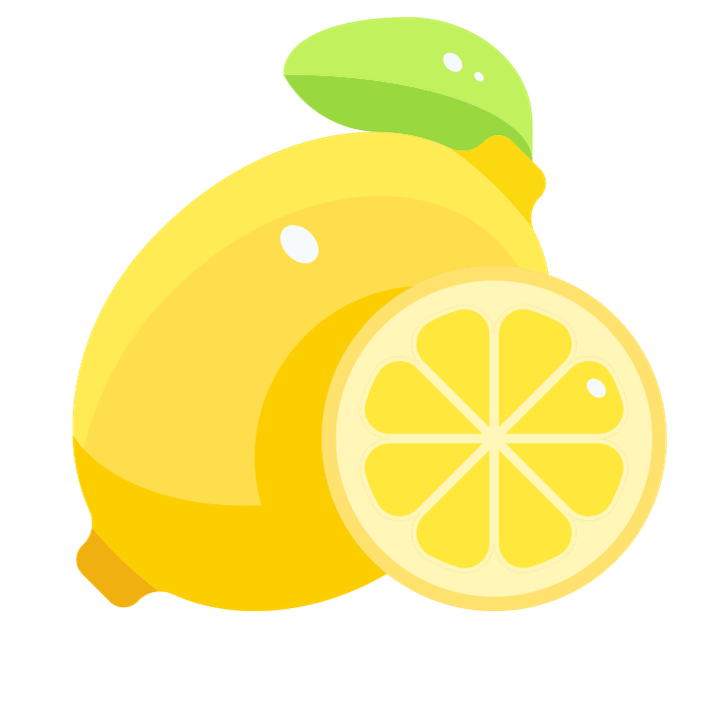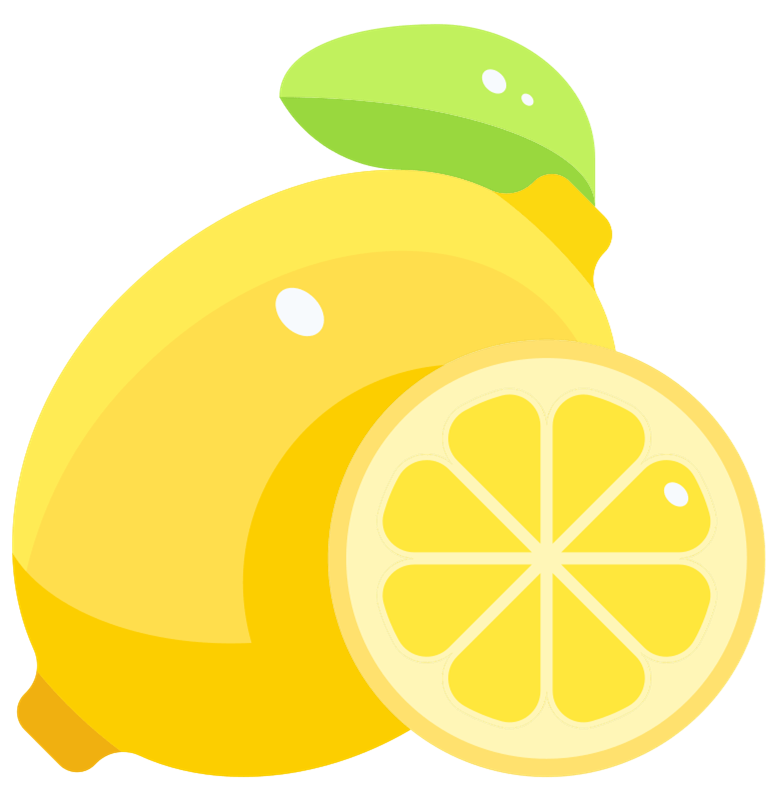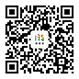# 【柠檬】ins潮流清新饮品销售黄绿

LEMON
~爱，柠檬~<<<<<<<<<<<<<~柠檬乌龙冻~LEMON<<<<<<<<<<<<<~柠檬乌龙冻~LEMON<<<<<<<<<<<<<~柠檬乌龙冻~LEMON<<<<<<<<<<<<<LEMON